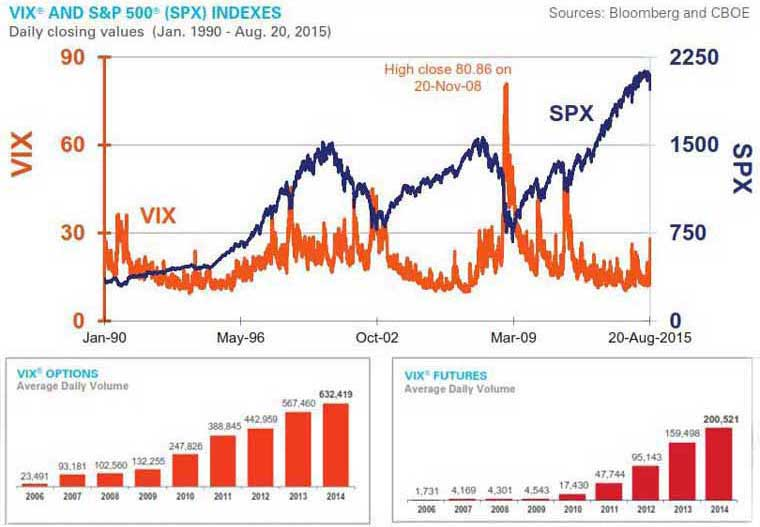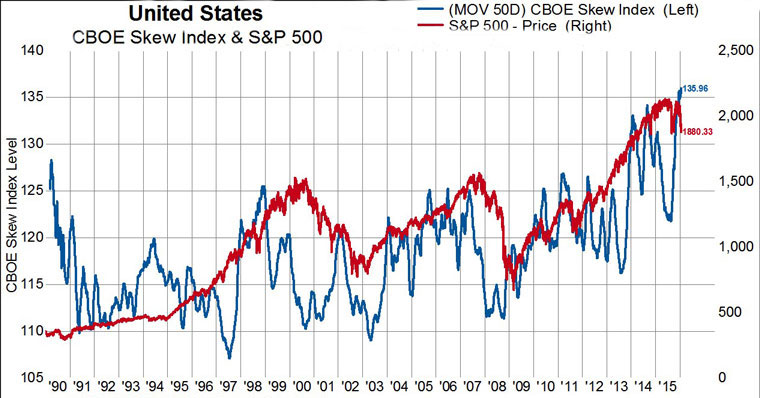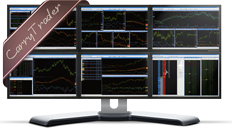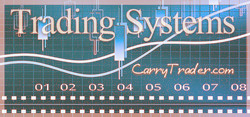Forecasting Market’s Volatility using two CBOE Volatility Indexes (VIX & Skew Index)

Options volatility indexes are used in forecasting future market volatility and the investor sentiment. The analysis of such volatility measures may assist investors managing and diversifying their portfolio more effectively.

In this analysis, two (2) important market volatility barometers are presented, both calculated based on the volatility of CBOE options:

• CBOE Volatility Index (^VIX)
• CBOE Skew Index (^SKEW)

The CBOE includes several other volatility indexes on stock indices, ETFs, shares, commodities, and tradable volatility contracts (VIX options, VIX futures, etc.). At the end of this analysis, you may find the full range of CBOE volatility indexes.

(1) CBOE Volatility Index (^VIX)

The CBOE Volatility Index (VIX) is considered the most important barometer of equity market volatility. The VIX Index is based on options contracts, on the S&P 500 Index (SPX). It is designed to reflect investors' consensus view of 30-day expected stock market volatility.

The Role of Options Volatility as a Forecasting Tool

The price of the call and put options can be used to calculate implied volatility because volatility is one of the factors used to calculate the value of these options. Any volatility modification of the underlying instrument makes an option contract more (or less) valuable, as there is a greater (or smaller) probability that the option may expire in-the-money, at the end of its maturity.

■ The Greater the Options Volatility → the Greater the Option Price -Therefore, other things being equal, a higher option price incurs greater volatility.

Note: The other two main factors when pricing an option, except volatility, include intrinsic value and time to maturity.VIX Interpretation

VIX is quoted in percentage points and translates, roughly, to the expected movement (with the assumption of a 68% likelihood i.e. one standard deviation) in the S&P 500 index over the next 30-day period, which is then annualized.

■ Example (based on CBOE guide):

If VIX is 15, this represents an expected annualized change, with a 68% probability, of less than 15% over the next 30 days; thus one can infer that the index options markets expect the S&P 500 to move up or down 15%/√12 = 4.33% over the next 30-day period. That is, index options are priced with the assumption of a 68% likelihood (one standard deviation) that the magnitude of the change in the S&P 500 in 30-days will be less than 4.33% (up or down).

VIX Calculation

The CBOE VIX Index is a volatility index comprised of options rather than stocks. This is the generalized formula used in the VIX calculation:(2) CBOE Skew Index (^SKEW)

CBOE Skew Index measures the implied volatility of deep out-of-the-money options. Deep out-of-the-money options are options contracts that are not profitable:

■ Deep out-of-the-money options, means a great difference between the Current Price and the Price of Execution of the Underlying Instrument. This difference is producing a highly Negative Intrinsic Value.

The CBOE Skew IndexSM or "SKEW" – is an option-based indicator that measures the perceived tail risk of the distribution of S&P 500 returns at a 30- day horizon.

CBOE Skew Interpretation

The CBOE Skew Index works as a measure of panic, in the Equity Markets. As concerns S&P500, there have been 15 times in the past when the CBOE Skew Index readings moved above 140, during the period 1990-2015. 12 of those 15 times have been in the last two years (2013-2015). An explanation for the increase of the Skew Index spikes is the increase in the cost of hedging during the past couple of years.SKEW Calculation

SKEW is derived from the price of S&P 500 skewness. That price is calculated from the prices of S&P 500 options using the same type of algorithm as for the CBOE Volatility Index (VIX).

SKEW typically ranges from 100 to 150. The skew value of 100 means that the perceived distribution of S&P 500 log-returns is normal and the probability of outlier returns is therefore negligible. As SKEW rises above 100, the left tail of the S&P 500 distribution acquires more weight, and the probabilities of outlier returns become more significant.

Other CBOE Volatility Indexes

The Chicago Board Options Exchange (CBOE) calculates and updates the values of about 30 indexes based on the expected volatility of different securities.

Volatility Indexes (Currency Futures)

Volatility Indexes (Interest Rates)

Volatility Indexes (Stock Indexes)

Volatility Indexes (ETFs)

Volatility Indexes (Single Stocks)

Volatility of VIX

 ■ COMPARE PROVIDERS ■ LEARNING RESOURCES

CBOE Volatility Index & CBOE Skew Index

Success in Currency Trading comes when experience meets perfect information...Focus constantly on improving your trading process and money will flow naturally:Trade the Global Markets using State-of-the-Art Technology...### Global MarketsSpread as low as 1.0 pip on EURUSD and free VPS Hosting..### Foreign Exchange

The Foreign Exchange Market is the largest Financial Market in the world with daily volumes of more than 4 trillion USD. When trading Forex you need a reliable and high-regulated Forex broker and a trading strategy that works:

(iii) Major Currency Pairs

### Global Markets

Trading the Financial Markets requires a perfect understanding of how demand and supply shift from time to time. Equities tend to follow bullish cycles lasting 7-9 years, and after, bearish cycles lasting 3-4 years. Energies tend to follow annual time patterns while Precious Metals tend to follow longer time cycles: